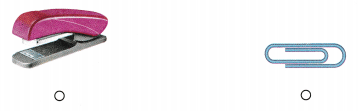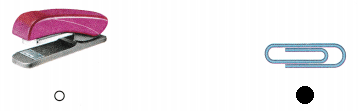# Texas Go Math Kindergarten Lesson 19.4 Answer Key Compare Weights

Refer to our Texas Go Math Kindergarten Answer Key Pdf to score good marks in the exams. Test yourself by practicing the problems from Texas Go Math Kindergarten Lesson 19.4 Answer Key Compare Weights.

## Texas Go Math Kindergarten Lesson 19.4 Answer Key Compare Weights

Essential Question
How can you compare the weights of two objects?

Explore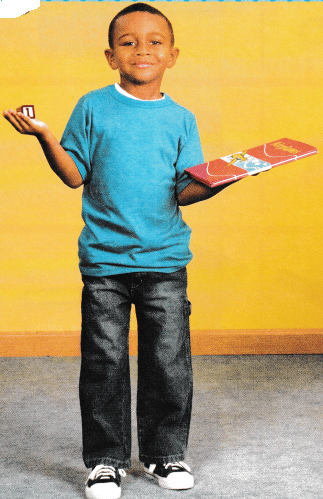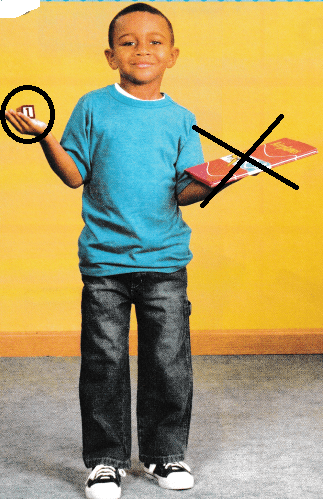Explanation:
In the right hand there is a cube
in the left hand there is a book
book is heavier than the cube.

Directions
Look at the picture. Compare the weights of the two objects. Use the words heavier than, lighter than, or about the same weight to describe the weights. Draw a circle around the lighter object. Draw an X on the heavier object.

Share and Show

Question 1.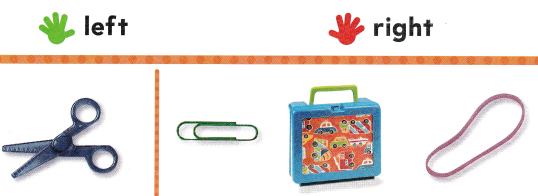Explanation:
The box is heavier than the scissors
so, circled the box.

Question 2.Explanation:
The book is lighter than the bag pack.
so, circled the bag pack.

Question 3.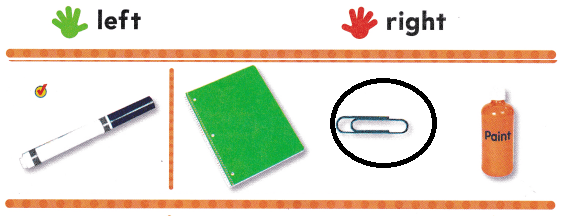Explanation:
The marker is heavier than the pin
so, circled the pin.

Question 4.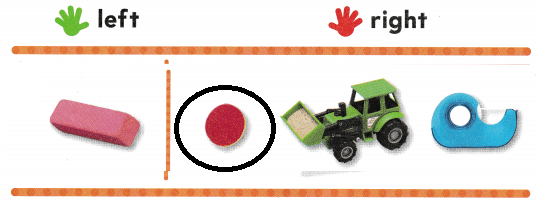Explanation:
The eraser is heavier than the ball
so, marked the ball.

Directions
Find the first object in the row, and hold it in your left bond. Find the rest of the objects in the row, and hold each object in your right hand. 1. Draw a circle around the object that is heavier than the object in your left hand. 2. Circle the object that is heavier than the object in your left hand. 3-4. Circle the object that is lighter than the object in your left hand

Question 5.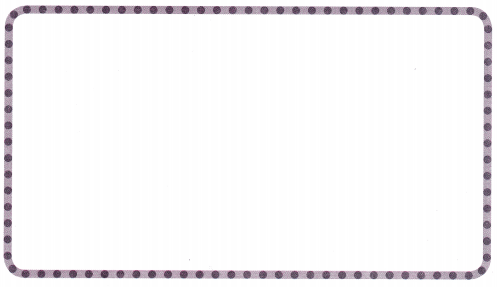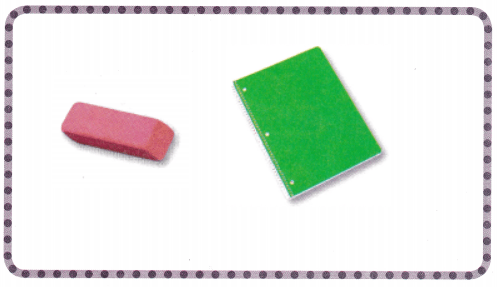Explanation:
The book is heavier than the book
In class room we found eraser and book

Question 6.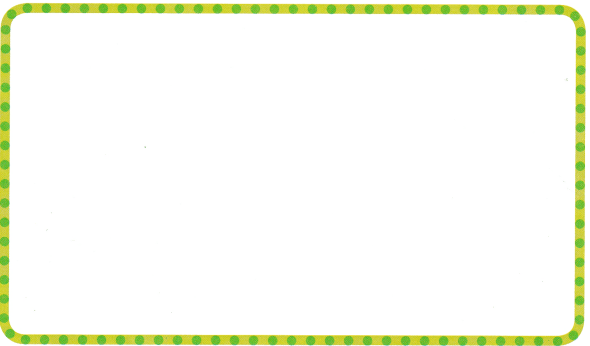Explanation:
In class room we found book and bag pack
bag pack is heavier than the book.

Directions
Find a book in the classroom. 5. Find a classroom object that is lighter than the book. Draw it in the work space. 6. Find a classroom object that is heavier than the book. Draw it in the work space.

Problem Solving

Question 7.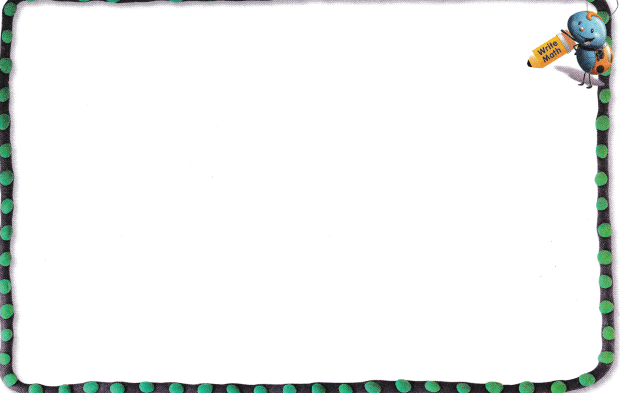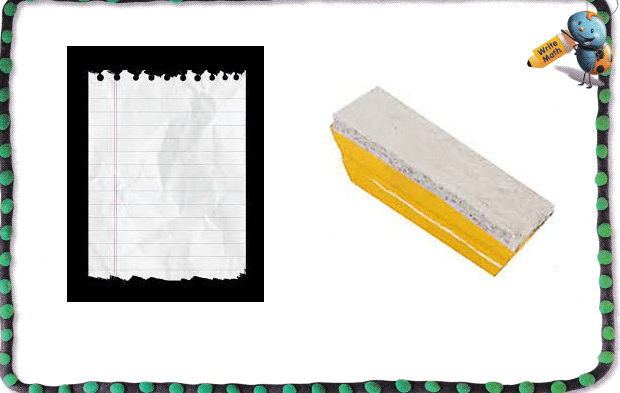Explanation:
The piece of paper is lighter than the duster
In class room we found paper and duster.

Question 8.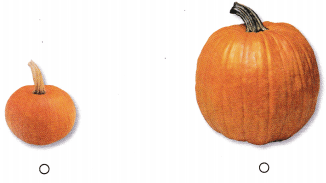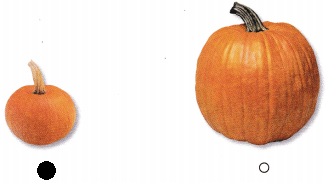Explanation:
The small pumpkin is lighter than the big pumpkin
so, marked the small pumpkin.

Directions
7. Draw to show what you know about comparing the weights of a piece of paper and another object Tell a friend about your drawing. 8. Choose the correct answer. Which pumpkin is lighter?

### Texas Go Math Kindergarten Lesson 19.4 Homework and Practice

Question 1.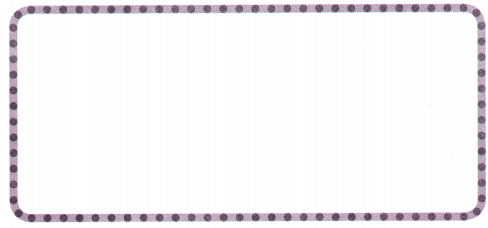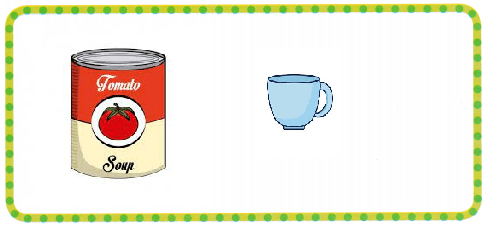Explanation:
In house hold we found tomato soup and the cup
tomato soup can is heavier than the cup.

Question 2.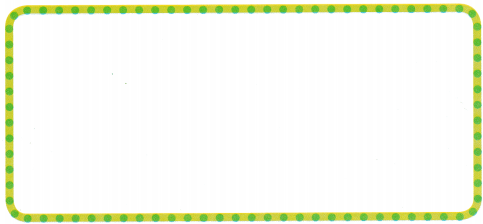Explanation:
The water melon is heavier than the knife
choose knife and water melon as the

Directions
Find a household object, such as a can of soup. 1. Find on object that is lighter than the household object. Draw it in the workspace. 2. Find an object that is heavier than the household object. Draw it in the workspace.

Texas Test prep

Lesson Check

Question 3.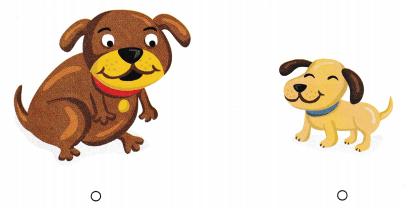Explanation:
The puppy is lighter than the dog
so, marked the puppy.

Question 4.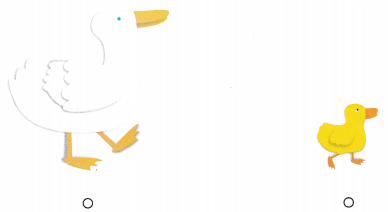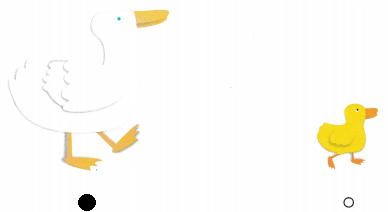Explanation:
The mommy duck is heavier that the baby duck
so, marked the baby duck.

Question 5.